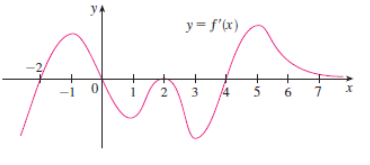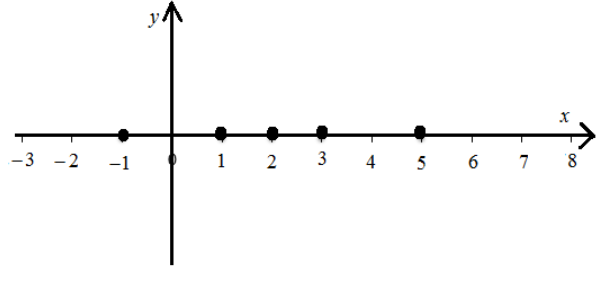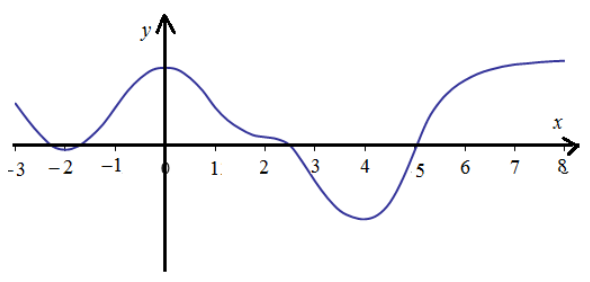# To graph: For the function f ' ' .### Single Variable Calculus: Concepts...

4th Edition
James Stewart
Publisher: Cengage Learning
ISBN: 9781337687805### Single Variable Calculus: Concepts...

4th Edition
James Stewart
Publisher: Cengage Learning
ISBN: 9781337687805

#### Solutions

Chapter 2, Problem 44RE

(a)

To determine

## To graph: For the function f'' .

Expert Solution

### Explanation of Solution

Given information:

The given graph of the derivative f' of f is shown in figure (1).Figure (1)

Graph:

The graph of f'' is positive when f' is increasing and negative when f' is negative.

All the local extremes, f''(x)=0 .

The plot for local extremes is shown in figure (2).Figure (2)

The plot for f'' is shown in figure (3).Figure (3)

Interpretation: Graph for the function f'' is shown in figure (3).

(b)

To determine

Expert Solution

### Explanation of Solution

Given information:

The given graph of the derivative f' of f is shown in figure (1).Figure (1)

Graph:

The function f is snit-derivative of f' . It has local extremes when f'(x)=0 .

While it is increases when f'(x)>0 and decreases when f'(x)<0 . Start fromleft and the graph of f is shown in figure (4).Figure (4)

Interpretation: Graph for the function f'' is shown in figure (4).

### Have a homework question?

Subscribe to bartleby learn! Ask subject matter experts 30 homework questions each month. Plus, you’ll have access to millions of step-by-step textbook answers!